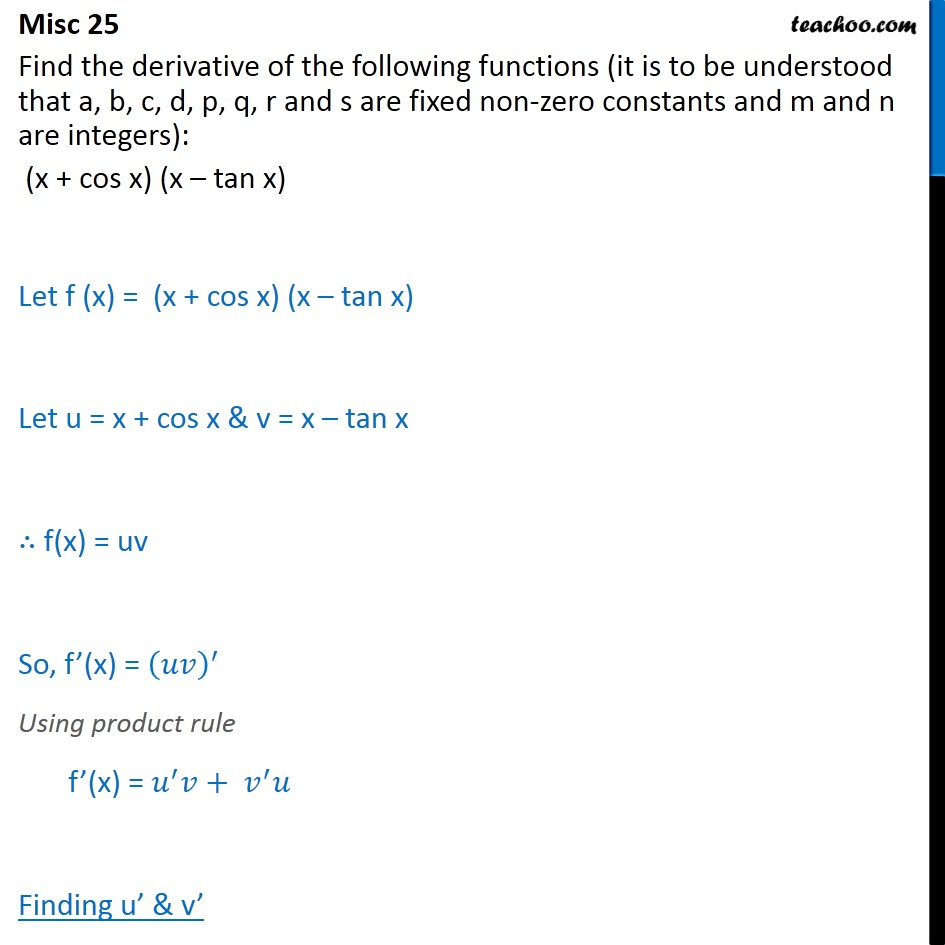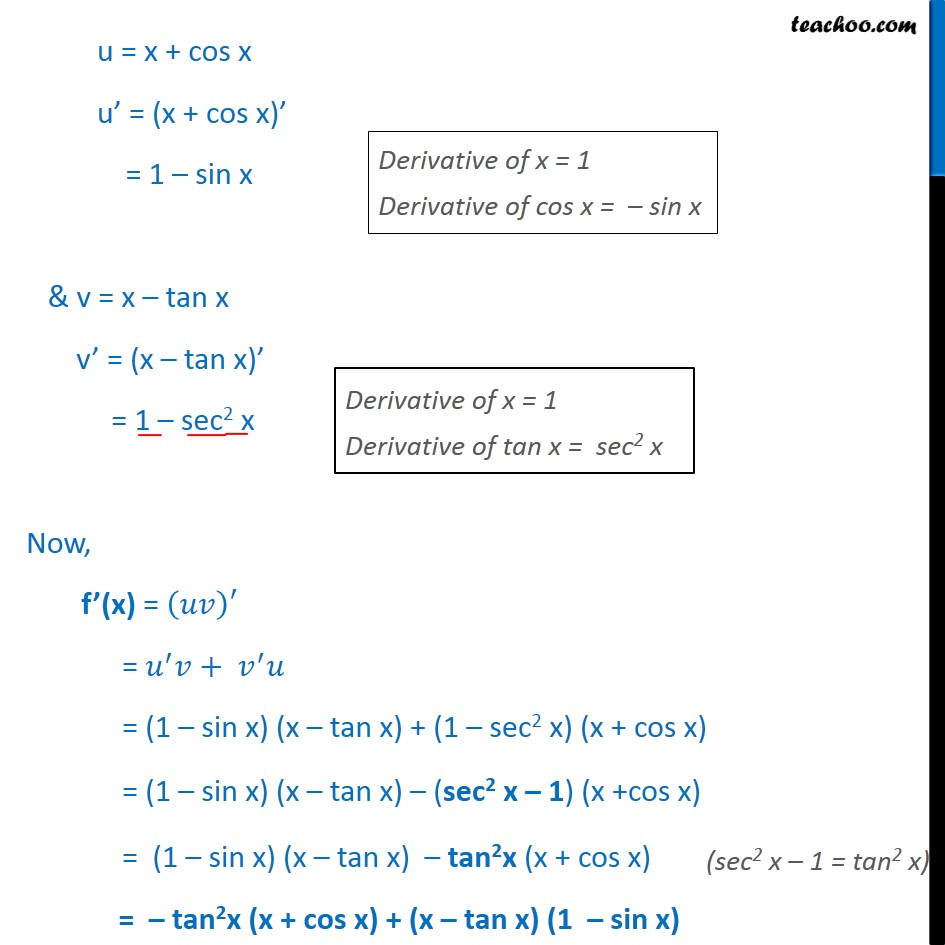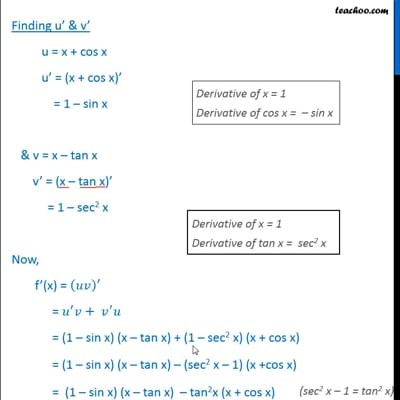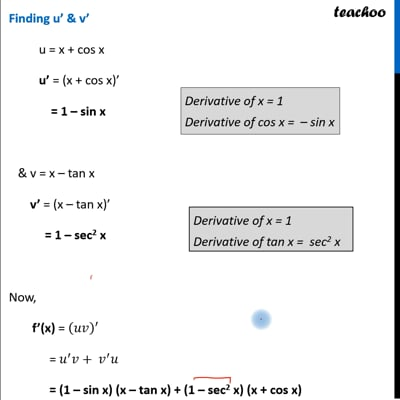Miscellaneous

Chapter 13 Class 11 Limits and Derivatives
Serial order wiseThis video is only available for Teachoo black usersThis video is only available for Teachoo black users

Get live Maths 1-on-1 Classs - Class 6 to 12

### Transcript

Misc 25 Find the derivative of the following functions (it is to be understood that a, b, c, d, p, q, r and s are fixed non-zero constants and m and n are integers): (x + cos x) (x tan x) Let f (x) = (x + cos x) (x tan x) Let u = x + cos x & v = x tan x f(x) = uv So, f (x) = Using product rule f (x) = + Finding u & v u = x + cos x u = (x + cos x) = 1 sin x & v = x tan x v = (x tan x) = 1 sec2 x Now, f (x) = = + = (1 sin x) (x tan x) + (1 sec2 x) (x + cos x) = (1 sin x) (x tan x) (sec2 x 1) (x +cos x) = (1 sin x) (x tan x) tan2x (x + cos x) = tan2x (x + cos x) + (x tan x) (1 sin x)